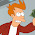## Friday, 22 May 2015

### Object.equals, primitive '==', and Arrays.equals ain't equal

It is a fact well known to those who know it well that "==" != "equals()" the example usually going something like:
String a = "Tom";
String b = new String(a);
-> a != b but a.equals(b)

It also seems reasonable therefore that:
String[] arr1 = {a};
String[] arr2 = {b};
-> arr1 != arr2 but Arrays.equals(arr1, arr2)

So far, so happy... That's examples for you...
For primitives we don't have the equals method, but we can try boxing:
int i = 0;
int j = 0;
-> i == j and also ((Integer)i).equals((Integer)j), and Arrays.equals({i}, {j})

### Floats ruin everything

But... some primitives are more equal then others. Consider for instance the following example:
float f1 = Float.Nan;
float f2 = Float.Nan;
-> f1 != f2, and also f1 != f1, Nan's are nasty

This is what floats are like children, they be treacherous little hobbitses and no mistake. The fun starts when you box them fuckers:
-> ((Float)f1).equals((Float)f2), because Java likes to fix shit up
-> Arrays.equals({((Float)f1)},{((Float)f2)}), hmm consistent
-> but also: Arrays.equals({f1},{f2})...

This is counter to how one would normally think arrays are compared. You would think that for primitives (skipping arrays null and length checks):
boolean feq = true;
for(int i=0;i<farr1.length;i++){
if(farr1[i] != farr2[i]) {
feq = false; break;
}
}
Is the same as:
boolean feq = Arrays.equals(farr1,farr2);
But for double[] and float[] the contract has been changed to accommodate Nan. This is perhaps a good decision on the JDK authors side, but it is somewhat surprising.
Conclusion: 2 arrays can be equal, even if some elements are not equal.

### Objects are weirdLet's consider objects for a second. We started with a != b does not predicate that !a.equals(b), but what about a == b?
The Object.equals() javadoc offers the following wisdom:
The equals method implements an equivalence relation on non-null object references:

• It is reflexive: for any non-null reference value x, x.equals(x) should return true.
• It is symmetric: for any non-null reference values x and y, x.equals(y) should return true if and only if y.equals(x) returns true.
• It is transitive: for any non-null reference values x, y, and z, if x.equals(y) returns true and y.equals(z) returns true, then x.equals(z) should return true.
• It is consistent: for any non-null reference values x and y, multiple invocations of x.equals(y) consistently return true or consistently return false, provided no information used in equals comparisons on the objects is modified.
• For any non-null reference value x, x.equals(null) should return false.
You know what the most important word in the above lines is? SHOULD
In the wonderful land of Should, all classes behave and if they bother implementing an equals method they follow the above rules (and also override the hashcode method while they are there).
But what happens when an object is implement perversely, like this:
public class Null {
@Override
public boolean equals(Object obj) {
return obj == null;
}
}

This has some nice implications:
Null n = new Null();
-> n != null, but n.equals(null)
Object[] a = {n};
Object[] b = {null};
Object[] c = {n};
-> Arrays.equals(a, b) is true, because n.equals(null)
-> Arrays.equals(b, a) is false, because null != n
-> Arrays.equals(a, a) is true, because a == a
-> Arrays.equals(a, c) is false, because !n.equals(n)

Or to quote The Dude: "Fuck it man, let's go bowling"

#### 1 comment:

1.LOL! Thanks, bro